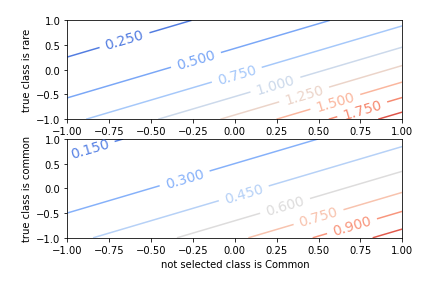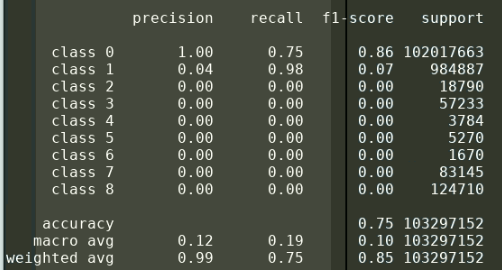# Passing the weights to CrossEntropyLoss correctly

I’m missing something. Loss (Error on rare class) same as Loss (Error on common class)?

weight_inbalance=torch.tensor([1000.0,1.0]) # very rare first class
loss_inbalance = torch.nn.CrossEntropyLoss(weight=weight_inbalance,reduction=‘mean’)

• batch of 2 samples, toggle only first sample
inbal_err_on_rare = loss_inbalance(torch.tensor([[-1.,-1],[-1,1]]), torch.tensor([0,1]))
inbal_err_on_com = loss_inbalance(torch.tensor([[1.,1],[-1,1]]), torch.tensor([0,1]))
print(inbal_err_on_rare, inbal_err_on_com)
tensor(0.6926) tensor(0.6926)

The same experiment on a ballance class yeilds the same equivalence, just offset in value.

weight_balance=torch.tensor([1.0,1.0])
loss_balance = torch.nn.CrossEntropyLoss(weight=weight_balance,reduction=‘mean’)
bal_err_on_not_rare = loss_balance(torch.tensor([[-1.,-1],[-1,1]]), torch.tensor([0,1]))
bal_err_on_com = loss_balance(torch.tensor([[1.,1],[-1,1]]), torch.tensor([0,1]))
print(bal_err_on_not_rare, bal_err_on_com)
tensor(0.4100) tensor(0.4100)

I generated the contours, and they look the same, just shifted.

weight_inbalance=torch.tensor([1000.0,1.0]) # very rare first class
weight_balance=torch.tensor([1.0,1.0])
loss_inbalance = torch.nn.CrossEntropyLoss(weight=weight_inbalance,reduction=‘mean’)
loss_balance = torch.nn.CrossEntropyLoss(weight=weight_balance,reduction=‘mean’)
X = np.arange(-1., 1.1, 0.1)
Y = np.arange(-1., 1.1, 0.1)
Z_inbalance = np.zeros((X.shape,Y.shape))
Z_balance = np.zeros((X.shape,Y.shape))
#print(X.shape, Y.shape, Z.shape)

i = -1
for x in X:
i+=1
j=-1
for y in Y:
j+=1
batch=torch.tensor([[x, y], [-1, 1]], dtype=torch.float32)

``````    X[i], Y[j], Z_inbalance[i, j] =x,y,loss_inbalance(batch, torch.tensor([0,1]))
X[i], Y[j], Z_balance[i, j] =x,y,loss_balance(batch, torch.tensor([0,1]))
``````

fig, ax = plt.subplots(2)

CS0 = ax.contour(Y,X,Z_inbalance,cmap=cm.coolwarm)
ax.clabel(CS0, inline=1, fontsize=14)
#ax.set_title(‘Inbalanced Classes’)
ax.set_ylabel(‘true class is rare’)
#ax.set_xlabel(“not selected class is Common”)

CS1 = ax.contour(Y,X,Z_balance,cmap=cm.coolwarm)
ax.clabel(CS1, inline=1, fontsize=14)
#ax.set_title(‘Balanced Classes’)
ax.set_ylabel(‘true class is common’)
ax.set_xlabel(“not selected class is Common”)

So either I am missing the point of weights, or made a newbie error. Either way, I would appreciate some comments.Note that in your first example both output tensors predict class1 only, so it’s expected to get the same loss value.
You can check it via:

``````torch.argmax(torch.tensor([[-1.,-1],[-1,1]]), 1)
> tensor([1, 1])

torch.argmax(torch.tensor([[1.,1],[-1,1]]), 1)
> tensor([1, 1])
``````

I haven’t looked into the second experiment as the formatting is unclear, but you could rerun it with another reduction (e.g. `reduction='sum'`), since the default `reduction='mean'` would normalize the loss with the applied weights.

Thank you, again.
Not following how the argmax relates CrossEntropyLoss. Please elaborate.

You are correct, somehow the “mean” nullifies the weight bias.

Experiment 1 shows an error on any logit when classes are balanced results in the same loss.
Experiment 2 shows if the rare class is the true class, the losses are amplified when any error occurs.

Experiment 1:

• balanced 2 classes,
• batch of 2 samples,
• introduce a single logit error and observe loss for true class = 0, 0 and 1, 1

loss = torch.nn.CrossEntropyLoss(weight=torch.tensor([1.,1.]),reduction=‘sum’)
print(“2 balanced class, reduction=sum”)

true_class_index = torch.tensor([0,0]) # so perfect prediction is [1,-1], [1,-1]
incorrect_sample_0_class_0 = loss(torch.tensor([[-1., -1],[1.,-1]]), true_class_index)
incorrect_sample_0_class_1 = loss(torch.tensor([[1., 1],[1.,-1]]), true_class_index)
incorrect_sample_1_class_0 = loss(torch.tensor([[1., -1],[-1.,-1]]), true_class_index)
incorrect_sample_1_class_1 = loss(torch.tensor([[1., -1],[1.,1]]), true_class_index)
print(f"all have one logit wrong {incorrect_sample_0_class_0:0.2f}, {incorrect_sample_0_class_1:0.2f}, {incorrect_sample_1_class_0:0.2f}, {incorrect_sample_1_class_1:0.2f}")

• all have one logit wrong 0.82, 0.82, 0.82, 0.82

true_class_index = torch.tensor([1,1]) # so perfect prediction is [1,-1], [1,-1]
incorrect_sample_0_class_0 = loss(torch.tensor([[1., 1],[-1.,1]]), true_class_index)
incorrect_sample_0_class_1 = loss(torch.tensor([[-1., -1],[-1.,1]]), true_class_index)
incorrect_sample_1_class_0 = loss(torch.tensor([[-1., 1],[1.,1]]), true_class_index)
incorrect_sample_1_class_1 = loss(torch.tensor([[-1., 1],[-1.,-1]]), true_class_index)
print(f"all have one logit wrong {incorrect_sample_0_class_0:0.2f}, {incorrect_sample_0_class_1:0.2f}, {incorrect_sample_1_class_0:0.2f}, {incorrect_sample_1_class_1:0.2f}")

• all have one logit wrong 0.82, 0.82, 0.82, 0.82

Experiment 2:

• unbalanced 2 classes,
• batch of 2 samples,
• introduce a single logit error and observe loss for true class = 0, 0 and 1, 1

loss = torch.nn.CrossEntropyLoss(weight=torch.tensor([10000.,1.]),reduction=‘sum’)
print(“2 unbalanced class, reduction=sum”)

true_class_index = torch.tensor([0,0]) # so perfect prediction is [1,-1], [1,-1]
incorrect_sample_0_class_0 = loss(torch.tensor([[-1., -1],[1.,-1]]), true_class_index)
incorrect_sample_0_class_1 = loss(torch.tensor([[1., 1],[1.,-1]]), true_class_index)
incorrect_sample_1_class_0 = loss(torch.tensor([[1., -1],[-1.,-1]]), true_class_index)
incorrect_sample_1_class_1 = loss(torch.tensor([[1., -1],[1.,1]]), true_class_index)
print(f"all have one logit wrong {incorrect_sample_0_class_0:0.2f}, {incorrect_sample_0_class_1:0.2f}, {incorrect_sample_1_class_0:0.2f}, {incorrect_sample_1_class_1:0.2f}")
> - all have one logit wrong 8200.75, 8200.75, 8200.75, 8200.75

true_class_index = torch.tensor([1,1]) # so perfect prediction is [1,-1], [1,-1]
incorrect_sample_0_class_0 = loss(torch.tensor([[1., 1],[-1.,1]]), true_class_index)
incorrect_sample_0_class_1 = loss(torch.tensor([[-1., -1],[-1.,1]]), true_class_index)
incorrect_sample_1_class_0 = loss(torch.tensor([[-1., 1],[1.,1]]), true_class_index)
incorrect_sample_1_class_1 = loss(torch.tensor([[-1., 1],[-1.,-1]]), true_class_index)
print(f"all have one logit wrong {incorrect_sample_0_class_0:0.2f}, {incorrect_sample_0_class_1:0.2f}, {incorrect_sample_1_class_0:0.2f}, {incorrect_sample_1_class_1:0.2f}")

• all have one logit wrong 0.82, 0.82, 0.82, 0.82

btw, is there a way to post code snippets and not loose indentation on this site?

An excellent summary for CrossEntropy https://youtu.be/ErfnhcEV1O8

Is it generally good practice to make weights linearly related to the number of related label pixels? ie. If you have 10 of class 1, 10 of class 2, and 20 of class 3, your weights would be [1,1,2]? I am facing a segmentation problem where there are many orders of magnitude difference between the each class and not sure what loss function/weights to handle this with.

2 Likes

Usually you increase the weight for minority classes, so that their loss also increases and forces the model to learn these samples. This could be done by e.g. the inverse class count (class frequency).

6 Likes

Hi @ptrblck,
I am looking into using multiple ignore_indices for loss computations. ignore_index argument expects an integer so I cannot use that.

Will it be equivalent to making the loss per pixel (for example) 0 for all those indices after the loss computation?

You could try to zero out the losses at all indices which should be ignored.
Would setting all these indices to a specific index value work as an alternative?
If so, you could still use the `ignore_index` argument and wouldn’t have to implement a manual approach.

Is there a paper on the use of weights to handle class imbalance? Can someone post a link to a reference?

I got crossentropyloss working without weights on a dataset with 98.8% unlabeled 1.1% labeled data and got relatively good results, so I assumed adding weights to the training would improve results. When I added weights the results were totally messed up, though. I made the weights inversely proportional to the label frequencies as well. ie. weights: [0.01172171 0.98827829].
Here is a gif showing: input labels, output of my crossentropyloss training without weights, output of my crossentropyloss training with weights (in that order)

Any ideas?

Kyle

How did you use `nn.CrossEntropyLoss` for unlabeled data? Did you skip the training samples without a target? Also, how should the `weight` counter this behavior?

Sorry my wording was confusing, by unlabelled I meant label 0, labeled meaning label 1. I hope this makes sense.

Thanks for the clarification.
How “good” were the original results, i.e. did you create the confusion matrix and checked the per-class accuracy or did you visually inspect the outputs?
I would assume that a prediction, which overfits on the majority class, might look quite good as these would cover 98% of all pixels.

Is your training and validation loss decreasing “properly” for the weighted use case or do you see that the model gets stuck?

This gif showing input labels, label results of training with no weights, then label results of training with weights was my visual inspection of the [0,1] label segmentations.

Here are results from my [0,1] label training with no weights- the one I considered ‘good’:And here are results from my [0,1] label training with weights inversely proportional to label frequency:This can be seen in the last frames of the gif where the label 1(green) is far overrepresented. You asked about loss for this training example. I checked, and it appears validation loss got to about 0.13 in the second epoch and never got better from there (training ended in 4th epoch at 0.15)

I also recently did a training example on a non binarized version of my data with no weights (labels [0,1…,8]) which had these results:Here the largest label was underrepresented and smaller structures went unrecognized. My ultimate goal is to have the multi-label segmentation work, I was just using a simplified version where labels [1,2,…8] were all set to 1 for testing purposes.

It may be of note that my ‘successful’ training ran for 6 epochs whereas the other two only went for 4. Not sure if this may be ‘convergence’ related.

Hello!

The link provided leads to a general documentation page, not a concrete passage.
The current documentation of the NLLLoss still generates a vague understanding of how the weights for classes are perceived.

For instance, as per text on this docspage, NLLLoss — PyTorch 1.8.1 documentation
the function is calculated from input with |C| rows whose values are purportedly softmax probabilities for each of the |C| classes. It is natural to suppose that the i_th element of the weights vector passed to
loss would match the values i_th column of input. But the torch NN modules do not explicitly indicate which final layer units (i.e. which columns of input to the loss) are related to which classes.
So which principle does torch utilise to map the weights onto classes in an arbitrary problem setup?

In my case, the weights are [0, 1] and after some trials it is now self-evident to me that the first element in weights pertains to class 0 and the second element pertains to class 1. But that’s probably the most simple case with the least ambiguity.

The reply of @done1892 in this post

gives an example for the classification problem with classes [0…4] and the author of the current post considered an example of classification with classes [0…10].
But here, the classes do not contain negative values and they are well ordered.
In a more general situation (e.g. with classes [-11, 0, 4]) would torch assign the weights to classes in an ascending manner, i.e. w1 to ‘’-11", w2 to “0”, w3 to “4”?

Thank you @ptrblck!
How can we ensure the mapping between labels and weights? e.g. if I have two classes (0 and 1) with class frequencies of 70% (class 0) and 30% (class 1), what should I choose between:

``````weights = torch.tensor([.7, .3])
``````

and

``````weights = torch.tensor([.3, .7])
``````

Thanks a lot in advanceThe `weights` are using the same class index, i.e. the loss is using `weight[class_index_of_sample]` to calculate the weighted loss. In your first example `class0` would get a weight of `0.7` while `class1` would use `0.3`.
In case your question was targeting the use case of “balancing” the loss given an imbalanced dataset, you should use the higher weight for the minority class, so the second approach.

1 Like

Yes, I would like to weight more the minority class, so [0.3, 0.7] would be the correct weighting.

But the question was more…

how can I be sure that the first weight value (0.3) gets assigned to class 0 and not to class 1?

Are the label values sorted in increasing order somewhere in the pipeline? Because maybe I have 6 samples with labels [1,0,0,0,1,0] and in this case the first label value would be 1.

thank you very much again

The order of weights corresponds to the class index values as described here:

For a target of `[1, 0, 0, 0, 1, 0]` the weights would then be indexed as `[0.7, 0.3, 0.3, 0.3, 0.7, 0.3]`.

Ok, thank you very much for clarifying!